# Alternating Current, Average Current, R.M.S. Value of Alternating Current

ALTERNATING CURRENT

Alternating Current is a form of the electric power generated and mostly used in the world. The magnitude of alternating current changes continuously with time and its direction is reversed periodically. It is represented by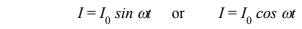where,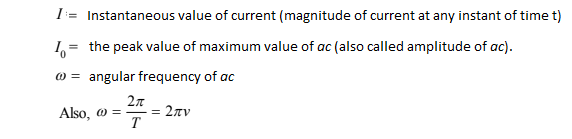where, T is the time period or period of ac It is equal to the time taken by the ac to go through one complete cycle of variation.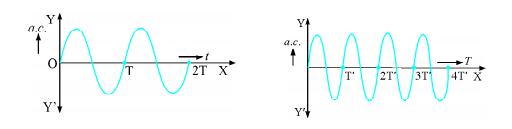The term used for ac holds equally for alternation voltage which may be represented by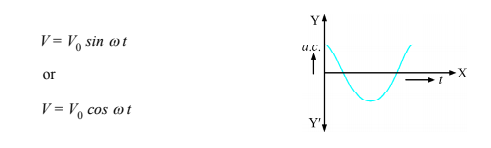In general,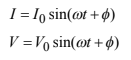Average Current (Mean Current)

As we know an alternating current is given byThe mean or average value of ac over any time t is given by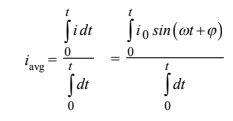In one complete cycle average current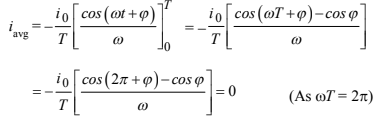Since ac is positive during the first half cycle and negative during the other half cycle so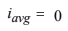Hence the dc instrument will indicate zero deflection when connected to a branch carrying ac current. So it is defined for either positive half cycle or negative half cycle.

Then, mean value of current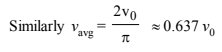R.M.S. Value of Alternating Current

The notation RMS refers to Root Mean Square, which is given by square root of mean of square current.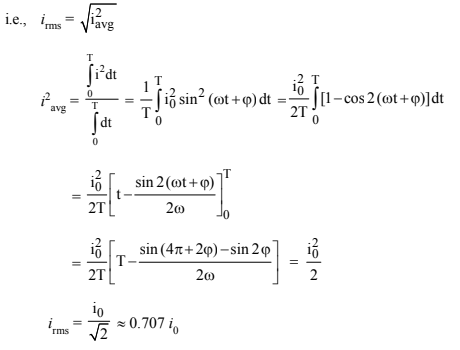Similarly, the RMS voltage is given by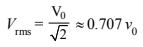The voltage across the resistor will be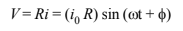The thermal energy developed in the resistor during the time t to t + dt is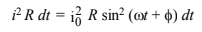The thermal energy developed in one-time period isSo in ac circuits, current and ac voltage are measured in terms of their rms values.

For eg. if house hold supply is 220V ac it means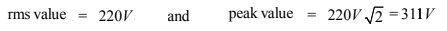Post By : Deepika Singh 25 Dec, 2018 2324 views Physics